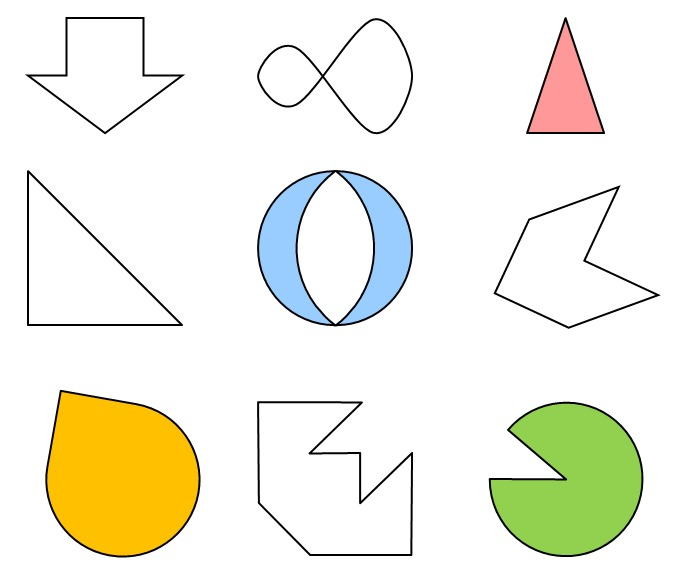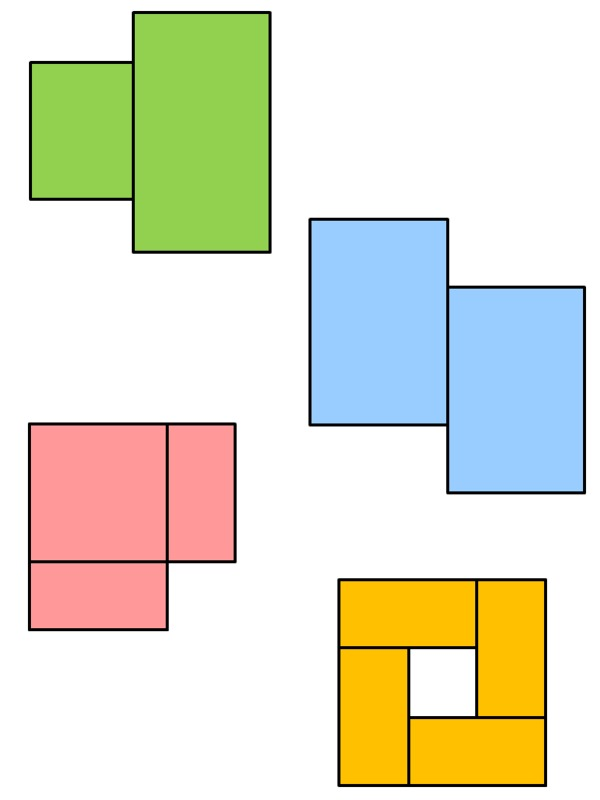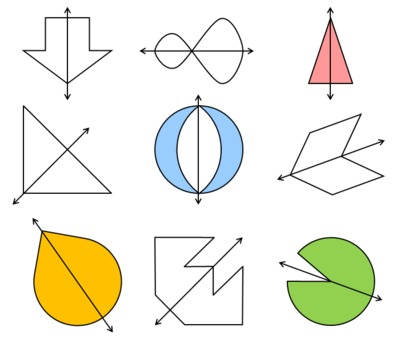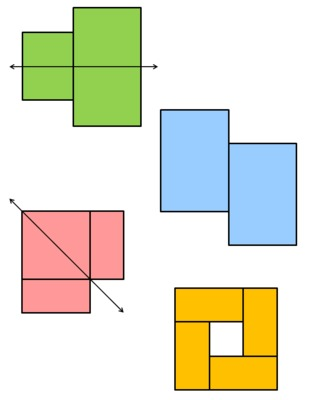# Finding Lines of Symmetry

Alignments to Content Standards: 4.G.A.3

1. Each shape below has a line of symmetry. Draw a line of symmetry for each shape.2. Not every shape has an line of symmetry. Which of the four shapes below have a line of symmetry? Draw a line of symmetry on them.3. Some shapes have many lines of symmetry. Draw all the lines of symmetry you can on the shape below. How many are there?## IM Commentary

The purpose of this task is for students to identify figures that have line symmetry and draw appropriate lines of symmetry. If students are first learning about symmetry, it would be good for them to create their own line-symmetric shapes by folding a piece of paper in half and cutting a shape out. Then they can darken the line represented by the fold to reinforce that it is a line of symmetry for their shape.

Identifying the lines of symmetry gets increasingly complex for the figures in part (a). Students are most likely to have difficulty when the lines of symmetry that have a diagonal orientation. The tricky thing about part (b) is that all the figures have symmetry, but only two have line symmetry (the other two figures have rotation symmetry). The fourth figure would have line symmetry if it weren't for the internal lines--this figure should produce a good conversation about what it means for figures to be symmetrical. In all of these cases, it would be very beneficial for students to have scissors and tracing paper so they can trace and cut the shapes out.

On part (c), students might connect opposite vertices or opposite sides but not both (getting an answer of 2 rather than 4). Students may also accidentally double their answer by counting how many “spokes” go from the center rather than how many complete lines there are.

This task includes an experimental GeoGebra worksheet, with the intent that instructors might use it to more interactively demonstrate the relevant content material. The file should be considered a draft version, and feedback on it in the comment section is highly encouraged, both in terms of suggestions for improvement and for ideas on using it effectively. The file can be run via the free online application GeoGebra, or run locally if GeoGebra has been installed on a computer.

## Attached Resources

• Printable PDF
• Geogebra File
• ## Solution

1.2.3.There are 4 lines of symmetry.